# College Chemistry : Identifying Redox Agents

## Example Questions

### Example Question #1 : Identifying Redox Agents

Consider the reaction shown below.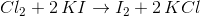Which of the following is true regarding this redox reaction?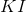is the reducing agent and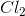is the oxidizing agentis the reducing agent andis the oxidizing agent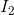is the reducing agent and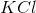is the oxidizing agentis the reducing agent andis the oxidizing agentis the reducing agent andis the oxidizing agent

Explanation:

For this question, we're given a reduction-oxidation (redox) reaction. We're asked to identify an answer choice that correctly states what the reducing agent is and what the oxidizing agent is.

Notice that another way we can write this reaction is by breaking up the ions as if they were dissociated in solution.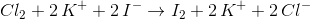Notice in the above example that the potassium ion remains in the same oxidation state. This is what is known as a spectator ion, meaning that it doesn't really participate in the reaction and remains unchanged on reactant and product sides of the equation. Hence, we can remove it from both sides to simplify things.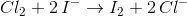In the simplified reaction shown above, we can see that the chlorine element is being reduced, while the iodine is being oxidized. Since the chlorine is being reduced, the iodine must be acting as the agent responsible for this. Hence, the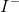is the reducing agent. Moreover, since the iodine is being oxidized, the chlorine must be the agent responsible for this. Thus,is the oxidizing agent.

Remember that thewe're referring to as the reducing agent is originally in the form of, which we can thus state as the reducing agent in the overall, unsimplified reaction.

### Example Question #2 : Identifying Redox Agents

Which compound is the reducing agent in this reaction?: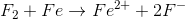There is none.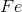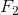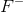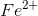Explanation:

A reducing agent is the compound in the reactants which becomes oxidized.

Oxidation is defined as the loss of electrons and is shown by the element changing to have a more positive oxidation number in the reaction.

To determine the reducing agent we simply see which compound is oxidized by determining the oxidation numbers of the elements in both the reactants and products, and comparing them.Reactants:

Forits oxidation state is simply zero because the oxidation number of an atom in its elemental form is always zero.

Same goes, which is why its oxidation number is also zero.

Products:has a charge of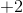, so its oxidation number is.has a charge of, so its oxidation number is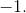Results:

Sinceloses electrons it is oxidized and therefore its the reducing agent.

None of the products can be the reducing agent because this reaction isn't reversible.

### Example Question #3 : Identifying Redox Agents

In this reaction, which compound is the reducing agent?: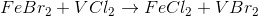This reaction isn't a redox reaction.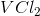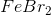This reaction isn't a redox reaction.

Explanation:

The reducing agent is the compound which contains the element being oxidized. Oxidation is defined as the loss of electrons, so the element which is oxidized becomes more positively charged.

In order to determine which element is oxidized we need to calculate the oxidation numbers of all the elements in the reactants and products and compare them. The element which has an oxidation number that increases overall is the one that is oxidized. We determine the oxidation numbers by applying the elementary rules of oxidation numbers to each compound.

For reference the chemical equation is: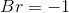because a rule of oxidation states is that oxidation number of all halogens is generally.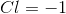for the same reason as bromine.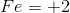becauseis neutrally charged and bromine has a charge ofand there are two bromines, so in order for the compound to maintain a neutral charge iron must have a charge of.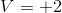for the same why iron is, except in this case it is due to chlorine'scharge instead of bromine.

Products:because the oxidation number of all halogens is generally.for the same reason as bromine.and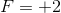because they both must be numbers that allow each of their respective compounds to be neutrally charged. For the same reasoning as in the reactants they both must be.

Since there are no changes in the oxidation numbers of any of these elements, this isn't a redox reaction therefore there is no reducing agent.

### Example Question #4 : Identifying Redox Agents

Which compound is the oxidizing agent?: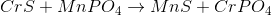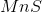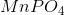This isn't a redox reaction.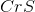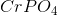Explanation:

The oxidizing agent is the compound which contains the element being reduced. Reduction is defined as gaining of electrons, so the element which is reduced becomes more negatively charged.

In order to determine which element is reduced we need to calculate the oxidation numbers of all the elements in the reactants and products and compare them. The element which has an oxidation number that decreases overall is the one that is reduced and therefore the oxidizing agent. We determine the oxidation numbers by applying the elementary rules of oxidation numbers to each compound.

For reference the chemical equation is: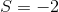because it is in the same group as oxygen which by the general rules of oxidation numbers has a charge of. Although sulfur can assume other oxidation numbers, it is alsoin this particular case because chromium is a transition metal that prefers to lose electrons to become more stable (become more positively charged) and since sulfur has a greater electronegativity it prefers a negatively charged state in this case.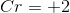because sulfur isand sinceis a neutral compound chromium must be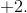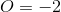because a general rule of oxidation numbers is that oxygen usually has an oxidation number of.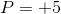In order to determine what phosphorus is we must look at the phosphate ion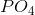. Phosphate is an anion which has a charge ofand in order for that to be possible with four oxygens (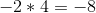) P must have a charge of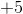.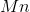=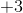because the phosphate anion has a charge of, somust offset that charge forto be neutrally charged.

Products:for the same reason it is in the reactants, except becauseis the transition metal in this case which prefers to lose electrons.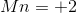becauseis neutrally charged, so it must offset the charge of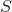.for the same reason as in the reactantsfor the same reason in the reactants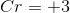to offset thecharge of the phosphate compound it is attached to.

Now let's compare the oxidation numbers of each element:

Oxygen, phosphate, and sulfur have the same oxidation states in both products and reactants.

Chromium increases from an oxidation state ofto.

Finally, manganese decreases from an oxidation state ofto, so it is the compound which is reduced because it gains electrons. Therefore the compound containing manganese is the oxidizing agent. This compound is, which is the right answer.